Binomial Probability
"Exactly", "At Most", "At Least
"

 Problem used for demonstration: A fair coin is tossed 100 times. What is the probability that: a.  heads will appear exactly 52 times? b.  there will be at most 52 heads? c.  there will be at least 48 heads?

 Dealing with "Exactly":

 A fair coin is tossed 100 times. What is the probability that: part a.  heads will appear exactly 52 times?

We have seen that the formula used with Bernoulli trials (binomial probability) computes the probability of obtaining exactly "r" events in "n" trials: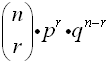where  n = number of trials,  r = number of specific events you wish to obtain,  p = probability that the event will occur,  and q = probability that the event will not occur (q = 1 - p, the complement of the event).

We have also seen that the built-in command binompdf (binomial probability density function) can also be used to quickly determine "exactly".

(Remember, the function binompdf is found under
DISTR (2nd VARS), arrow down to #0 binompdf
and the parameters are:
binompdf (number of trials, probability of occurrence, number of specific events)

binompdf (n, p, r)

Here is our answer to part a.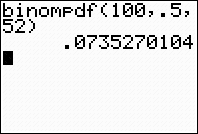If you want more information at a glance, this command can also be used to produce a list of the exact probabilities.

 Prepare two lists. In L1 place the numbers from 0 to 100 for easy reference.  The built-in sequence command will quickly fill L1 from 0 to 100.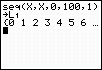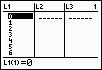The format for seq:  expression, variable,  starting value, ending value, increment. In L2, prepare the "exact" probabilities using binompdf,  using only the number of trials and the probability of occurrence.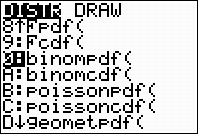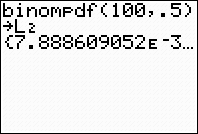(This is one method of filling a list.) The lists now contain, for 100 trials, the number of specific events (heads) in L1 and the exact probability of occurrence in L2.  Notice that our answer for exactly 52 heads remains the same.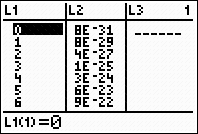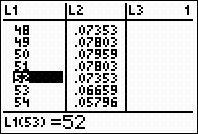Dealing with "At Most":

 A fair coin is tossed 100 times. What is the probability that: part b.  there will be at most 52 heads?

The formula needed for answering part b is :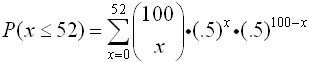There is a built-in command binomcdf (binomial cumulative density function) that can be used to quickly determine "at most".  Because this is a "cumulative" function, it will find the sum of all of the probabilities up to, and including, the given value of 52.

(The function binomcdf is found under
DISTR (2nd VARS), arrow down to #A binomcdf
and the parameters are:
binomcdf (number of trials, probability of occurrence, number of specific events)

binomcdf (n, p, r)

Here is our answer to part b.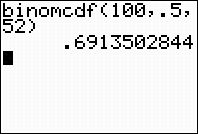Again, if you want more information at a glance regarding cumulative probabilities, this command can be used to produce a list of the "at most" probabilities.

 Prepare two lists. In L1 place the numbers from 0 to 100 for easy reference.  The built-in sequence command will quickly fill L1 from 0 to 100.The format for seq:  expression, variable,  starting value, ending value, increment. In L2, prepare the "at most" (cumulative) probabilities using binomcdf,  using only the number of trials and the probability of occurrence.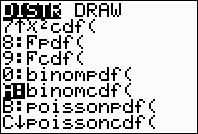(Method shown below is another method of filling a list.)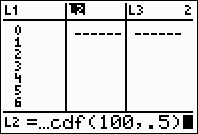Notice that our answer remains the same.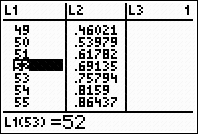Dealing with "At Least":

 A fair coin is tossed 100 times. What is the probability that: part c.  there will be at least 48 heads?

The formula needed for answering part b is :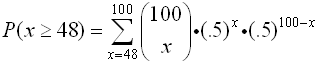Keep in mind that "at least" 48 is the complement of "at most" 47.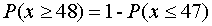In a binomial distribution,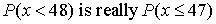.

While there is no built-in command for "at least", you can quickly find the result by creating this complement situation by subtracting from 1.  Just remember to adjust the value to 47.

(Remember, the function binomcdf is found under
DISTR (2nd VARS), arrow down to #A binomcdf.)

The adjusted formula for "at least" is 1 - binomcdf (n, p
, r - 1).

 Here is our answer to part c.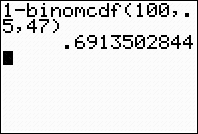The fact that this answer is the same as the "at most" answer for the number 52, is due to the symmetric nature of the distribution about its mean of 50.

Again, if you want more information at a glance regarding cumulative probabilities, this subtraction process can be used to produce a list of the "at least" probabilities.

 Prepare two lists. In L1 place the numbers from 0 to 100 for easy reference.  The built-in sequence command will quickly fill L1 from 0 to 100.The format for seq:  expression, variable,  starting value, ending value, increment. In L2, prepare the "at least" (1 - binomcdf) probabilities,  using as parameters the number of trials, the probability of occurrence, and one less than the value in L1.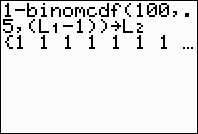Notice that our answer is the listing for 48.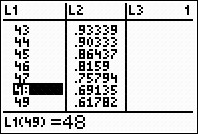Finding Your Way Around TABLE of  CONTENTS